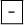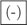Numbers, units and arithmetic

This free course is available to start right now. Review the full course description and key learning outcomes and create an account and enrol if you want a free statement of participation.

Free course

# 1.10 Negative number notation

It is worth mentioning notation at this point. You may have noticed that the minus sign used to denote a negative number is shorter, closer to the number and raised, compared with the minus sign used to denote subtraction. It is important to distinguish between the two, and it can help to think of 3, say, as ‘negative 3’ rather than ‘minus 3’. In the calculation 8 − 3 (8 subtract 3), the minus sign is an operator, an instruction to subtract; in 3, the sign is part of the number. The two types of minus sign are distinguished on many calculators. If you have a calculator handy, look at the key pad to see if you have two keys. They are often labelledand.

MU120_4M1